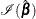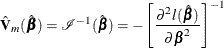### Newton-Raphson Method

Let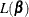be one of the likelihood functions described in the previous subsections. Let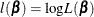. Findingsuch thatis maximized is equivalent to finding the solution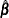to the likelihood equations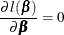With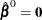as the initial solution, the iterative scheme is expressed as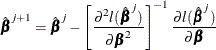The term after the minus sign is the Newton-Raphson step. If the likelihood function evaluated at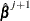is less than that evaluated at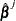, thenis recomputed using half the step size. The iterative scheme continues until convergence is obtained—that is, until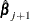is sufficiently close to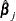. Then the maximum likelihood estimate ofis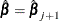.

The model-based variance estimate ofis obtained by inverting the information matrix